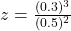## Electric charge is uniformly distributed inside a nonconducting sphere of radius 0.30 m. The electric field at a point P, which is 0.50 m fr

Question

Electric charge is uniformly distributed inside a nonconducting sphere of radius 0.30 m. The electric field at a point P, which is 0.50 m from the center of the sphere, is 15,000 N/C and is directed radially outward. At what distance from the center of the sphere does the electric field have the same magnitude as it has at P?

in progress 0
6 months 2021-07-19T00:49:11+00:00 1 Answers 99 views 0

## Answers ( )

The point that would have the same electric field as P is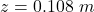from the center of the sphere.

Explanation:

From the question we are told that

The  radius of the sphere is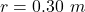The Electric field at point P is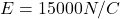The distance of point P from the center is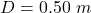Since the electric is directed radially outward it mean this it would be felt both inside and outside the sphere

The Electric field inside the sphere at a distance z is mathematically represented as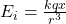where k is the coulomb’s constant with a  value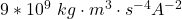q is the charge

The Electric field inside the sphere at a distance D  is mathematically represented as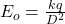To obtain the point of equal electric field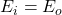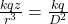We have that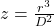Substituting values